# Understanding Tangents and Normals with Wolfram|Alpha

January 12, 2012 —Comments Off

Finding the tangents and normals of a mathematical function or relation is one of the most common exercises in any calculus course. In this post, I’ll show you the newest functionality in Wolfram|Alpha for discovering and investigating them.

The simplest example of a tangent is the “tangent line” to a one-dimensional curve in the plane. Graphically, the tangent line is a line that “just touches” the curve at some point, so that if it were moved just slightly, this one point of contact would become two.

If you ask Wolfram|Alpha for the tangent line of a specific function and point, it gives it in both graphical and algebraic/numerical form:

Notice that near the point of tangency, the line and the curve are nearly identical; the line does almost exactly what the curve does near that point. This is exactly why tangents are important: it’s often much easier to answer questions about linear functions, and tangents provide a way of approximating a complicated relationship with a linear relationship (a line or a plane). It’s also why the tangent is called the “linearization” or “linear approximation” of the relation.

For example, suppose you need to know the square root of 3.9. A very rough estimate would be 2, since 3.9 is roughly 4, and 2 = sqrt(4). Even better would be to linearize the function y = sqrt(x) at the point x = 4 and use this to find an estimate: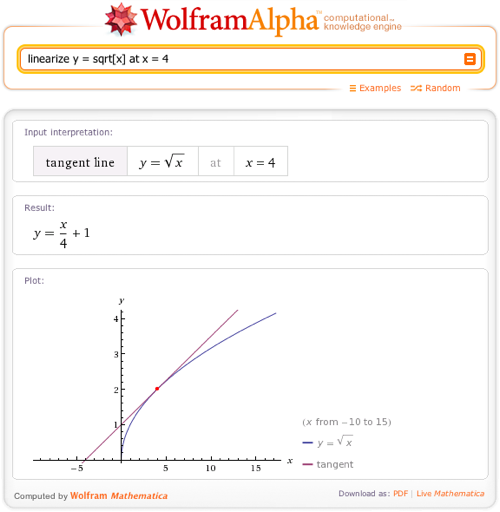This says that the linear approximation to sqrt(x) at x = 4 is x/4 + 1. Plugging x = 3.9 into this approximation yields 3.9/4 + 1 = 1.975, which is pretty close to the actual value of sqrt(3.9), an irrational number whose first six digits are 1.97484.

You can also ask Wolfram|Alpha for the slope of a tangent line to a function at a point, which is another common calculus question (it’s actually equal to the derivative of the function at that point).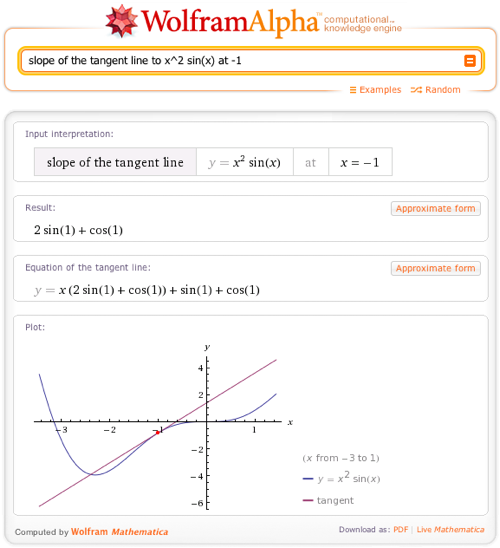What about higher-dimensional functions? A function or relation with two degrees of freedom is visualized as a surface in space, the tangent to which is a plane that just touches the surface at a single point. For example, here’s the tangent plane to z = sin[xy] at x = 1, y = .9, as displayed by Wolfram|Alpha: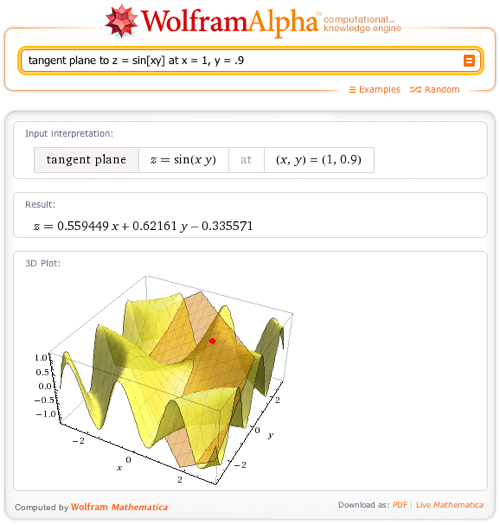The “normal” to a curve or surface is a kind of the complement of the tangent. The “normal line” to a one-dimensional curve is perpendicular to the tangent line and goes through the same point on the curve: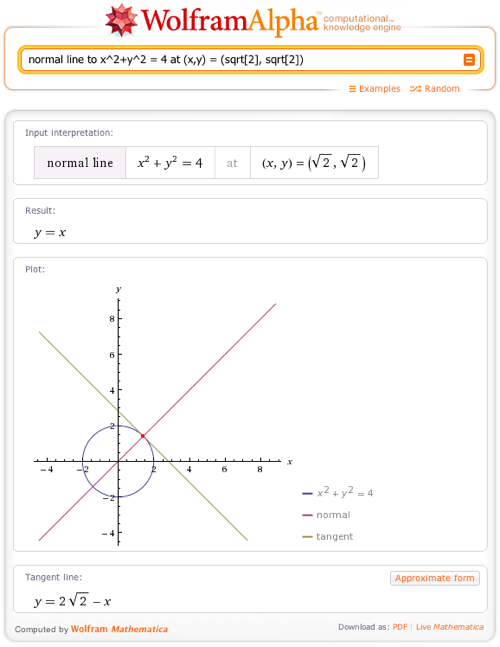The normal to a surface in space is also a line. It is the unique line that is perpendicular to the tangent plane at that point: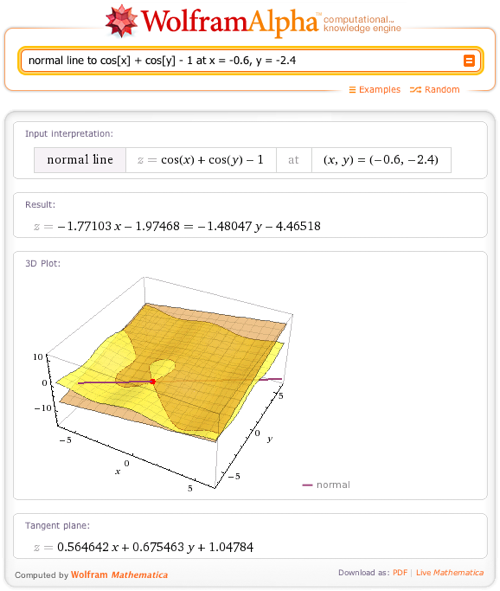Tangents and normals to higher-dimensional surfaces exist as well. Of course, no plot is possible, but Wolfram|Alpha will give you algebraic and numerical representations of the tangent and normal to any multidimensional surface and any point: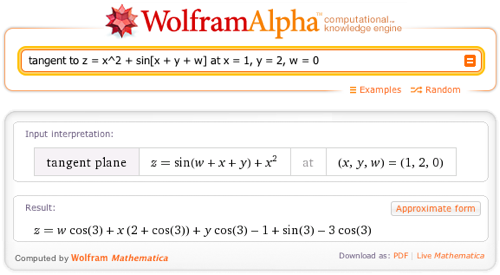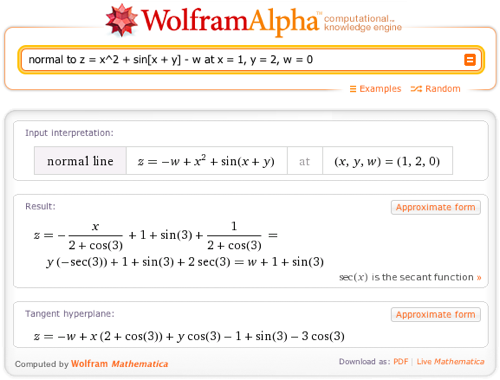I hope that this new functionality will be used by both students to check their understanding and by professionals to solve practical problems. Enjoy!